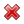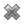# Fight Finance

#### CoursesTagsRandomAllRecentScoresScoreskeithphw $6,011.61 Jade$1,815.80 Boeti $1,002.09 ZOE HY$860.33 Chu $789.98 royal ne...$750.00 Leehy $713.33 Visitor$650.00 JennyLI $625.61 Visitor$590.00 Visitor $555.33 Visitor$550.00 Visitor $550.00 Visitor$540.00 Visitor $500.00 Yizhou$489.18 Visitor $480.00 Visitor$480.00 Visitor $470.00 Visitor$464.70

There are many ways to calculate a firm's free cash flow (FFCF), also called cash flow from assets (CFFA). Some include the annual interest tax shield in the cash flow and some do not.

Which of the below FFCF formulas include the interest tax shield in the cash flow?

$$(1) \quad FFCF=NI + Depr - CapEx -ΔNWC + IntExp$$ $$(2) \quad FFCF=NI + Depr - CapEx -ΔNWC + IntExp.(1-t_c)$$ $$(3) \quad FFCF=EBIT.(1-t_c )+ Depr- CapEx -ΔNWC+IntExp.t_c$$ $$(4) \quad FFCF=EBIT.(1-t_c) + Depr- CapEx -ΔNWC$$ $$(5) \quad FFCF=EBITDA.(1-t_c )+Depr.t_c- CapEx -ΔNWC+IntExp.t_c$$ $$(6) \quad FFCF=EBITDA.(1-t_c )+Depr.t_c- CapEx -ΔNWC$$ $$(7) \quad FFCF=EBIT-Tax + Depr - CapEx -ΔNWC$$ $$(8) \quad FFCF=EBIT-Tax + Depr - CapEx -ΔNWC-IntExp.t_c$$ $$(9) \quad FFCF=EBITDA-Tax - CapEx -ΔNWC$$ $$(10) \quad FFCF=EBITDA-Tax - CapEx -ΔNWC-IntExp.t_c$$

The formulas for net income (NI also called earnings), EBIT and EBITDA are given below. Assume that depreciation and amortisation are both represented by 'Depr' and that 'FC' represents fixed costs such as rent.

$$NI=(Rev - COGS - Depr - FC - IntExp).(1-t_c )$$ $$EBIT=Rev - COGS - FC-Depr$$ $$EBITDA=Rev - COGS - FC$$ $$Tax =(Rev - COGS - Depr - FC - IntExp).t_c= \dfrac{NI.t_c}{1-t_c}$$

 Project Data Project life 2 yrs Initial investment in equipment $600k Depreciation of equipment per year$250k Expected sale price of equipment at end of project $200k Revenue per job$12k Variable cost per job $4k Quantity of jobs per year 120 Fixed costs per year, paid at the end of each year$100k Interest expense in first year (at t=1) $16.091k Interest expense in second year (at t=2)$9.711k Tax rate 30% Government treasury bond yield 5% Bank loan debt yield 6% Levered cost of equity 12.5% Market portfolio return 10% Beta of assets 1.24 Beta of levered equity 1.5 Firm's and project's debt-to-equity ratio 25%

Notes

1. The project will require an immediate purchase of $50k of inventory, which will all be sold at cost when the project ends. Current liabilities are negligible so they can be ignored. Assumptions • The debt-to-equity ratio will be kept constant throughout the life of the project. The amount of interest expense at the end of each period has been correctly calculated to maintain this constant debt-to-equity ratio. Note that interest expense is different in each year. • Thousands are represented by 'k' (kilo). • All cash flows occur at the start or end of the year as appropriate, not in the middle or throughout the year. • All rates and cash flows are nominal. The inflation rate is 2% pa. • All rates are given as effective annual rates. • The 50% capital gains tax discount is not available since the project is undertaken by a firm, not an individual. What is the net present value (NPV) of the project? The US firm Google operates in the online advertising business. In 2011 Google bought Motorola Mobility which manufactures mobile phones. Assume the following: • Google had a 10% after-tax weighted average cost of capital (WACC) before it bought Motorola. • Motorola had a 20% after-tax WACC before it merged with Google. • Google and Motorola have the same level of gearing. • Both companies operate in a classical tax system. You are a manager at Motorola. You must value a project for making mobile phones. Which method(s) will give the correct valuation of the mobile phone manufacturing project? Select the most correct answer. The mobile phone manufacturing project's: Which one of the following will have no effect on net income (NI) but decrease cash flow from assets (CFFA or FFCF) in this year for a tax-paying firm, all else remaining constant? Remember: $$NI=(Rev-COGS-FC-Depr-IntExp).(1-t_c )$$ $$CFFA=NI+Depr-CapEx - ΔNWC+IntExp$$ Your friend is trying to find the net present value of a project. The project is expected to last for just one year with: • a negative cash flow of -$1 million initially (t=0), and
• a positive cash flow of $1.1 million in one year (t=1). The project has a total required return of 10% pa due to its moderate level of undiversifiable risk. Your friend is aware of the importance of opportunity costs and the time value of money, but he is unsure of how to find the NPV of the project. He knows that the opportunity cost of investing the$1m in the project is the expected gain from investing the money in shares instead. Like the project, shares also have an expected return of 10% since they have moderate undiversifiable risk. This opportunity cost is $0.1m $(=1m \times 10\%)$ which occurs in one year (t=1). He knows that the time value of money should be accounted for, and this can be done by finding the present value of the cash flows in one year. Your friend has listed a few different ways to find the NPV which are written down below. (I) $-1m + \dfrac{1.1m}{(1+0.1)^1}$ (II) $-1m + \dfrac{1.1m}{(1+0.1)^1} - \dfrac{1m}{(1+0.1)^1} \times 0.1$ (III) $-1m + \dfrac{1.1m}{(1+0.1)^1} - \dfrac{1.1m}{(1+0.1)^1} \times 0.1$ (IV) $-1m + 1.1m - \dfrac{1.1m}{(1+0.1)^1} \times 0.1$ (V) $-1m + 1.1m - 1.1m \times 0.1$ Which of the above calculations give the correct NPV? Select the most correct answer. For a price of$102, Andrea will sell you a share which just paid a dividend of $10 yesterday, and is expected to pay dividends every year forever, growing at a rate of 5% pa. So the next dividend will be $10(1+0.05)^1=10.50$ in one year from now, and the year after it will be $10(1+0.05)^2=11.025$ and so on. The required return of the stock is 15% pa. Would you like to the share or politely ? A very low-risk stock just paid its semi-annual dividend of$0.14, as it has for the last 5 years. You conservatively estimate that from now on the dividend will fall at a rate of 1% every 6 months.

If the stock currently sells for $3 per share, what must be its required total return as an effective annual rate? If risk free government bonds are trading at a yield of 4% pa, given as an effective annual rate, would you consider buying or selling the stock? The stock's required total return is: Economic statistics released this morning were a surprise: they show a strong chance of consumer price inflation (CPI) reaching 5% pa over the next 2 years. This is much higher than the previous forecast of 3% pa. A vanilla fixed-coupon 2-year risk-free government bond was issued at par this morning, just before the economic news was released. What is the expected change in bond price after the economic news this morning, and in the next 2 years? Assume that: • Inflation remains at 5% over the next 2 years. • Investors demand a constant real bond yield. • The bond price falls by the (after-tax) value of the coupon the night before the ex-coupon date, as in real life. Which of the following investable assets are NOT suitable for valuation using PE multiples techniques? A residential investment property has an expected nominal total return of 8% pa and nominal capital return of 3% pa. Inflation is expected to be 2% pa. All rates are given as effective annual rates. What are the property's expected real total, capital and income returns? The answer choices below are given in the same order. All things remaining equal, according to the capital asset pricing model, if the systematic variance of an asset increases, its required return will increase and its price will decrease. If the idiosyncratic variance of an asset increases, its price will be unchanged. What is the relationship between the price of a call or put option and the total, systematic and idiosyncratic variance of the underlying asset that the option is based on? Select the most correct answer. Call and put option prices increase when the:  Project Data Project life 1 year Initial investment in equipment$8m Depreciation of equipment per year $8m Expected sale price of equipment at end of project 0 Unit sales per year 4m Sale price per unit$10 Variable cost per unit $5 Fixed costs per year, paid at the end of each year$2m Interest expense in first year (at t=1) $0.562m Corporate tax rate 30% Government treasury bond yield 5% Bank loan debt yield 9% Market portfolio return 10% Covariance of levered equity returns with market 0.32 Variance of market portfolio returns 0.16 Firm's and project's debt-to-equity ratio 50% Notes 1. Due to the project, current assets will increase by$6m now (t=0) and fall by $6m at the end (t=1). Current liabilities will not be affected. Assumptions • The debt-to-equity ratio will be kept constant throughout the life of the project. The amount of interest expense at the end of each period has been correctly calculated to maintain this constant debt-to-equity ratio. • Millions are represented by 'm'. • All cash flows occur at the start or end of the year as appropriate, not in the middle or throughout the year. • All rates and cash flows are real. The inflation rate is 2% pa. All rates are given as effective annual rates. • The project is undertaken by a firm, not an individual. What is the net present value (NPV) of the project? You just bought a residential apartment as an investment property for$500,000.

You intend to rent it out to tenants. They are ready to move in, they would just like to know how much the monthly rental payments will be, then they will sign a twelve-month lease.

You require a total return of 8% pa and a rental yield of 5% pa.

What would the monthly paid-in-advance rental payments have to be this year to receive that 5% annual rental yield?

Also, if monthly rental payments can be increased each year when a new lease agreement is signed, by how much must you increase rents per year to realise the 8% pa total return on the property?

Ignore all taxes and the costs of renting such as maintenance costs, real estate agent fees, utilities and so on. Assume that there will be no periods of vacancy and that tenants will promptly pay the rental prices you charge.

Note that the first rental payment will be received at t=0. The first lease agreement specifies the first 12 equal payments from t=0 to 11. The next lease agreement can have a rental increase, so the next twelve equal payments from t=12 to 23 can be higher than previously, and so on forever.